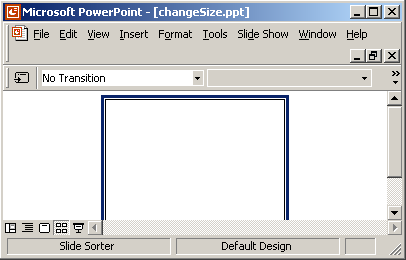Tutorials

# Change Size of PowerPoint Slide Using Java

In this example we are going to create a slide then change the size of the slide.

In this example we are going to create a slide then change the size of the slide.

# Change Size of PowerPoint Slide Using Java

In this example we are going to create a slide then change the size of the slide.

setPageSize(java.awt.Dimension pgsize):
This method is used to change the size current page. The size of page is passed as parameter in this method. The return type is void. This method is defined as public in SlideShow class.

The code of the program is given below:

 ``` import org.apache.poi.hslf.HSLFSlideShow; import org.apache.poi.hslf.model.Slide; import org.apache.poi.hslf.usermodel.SlideShow; import java.io.*; class changeSlideSize{   public static void main(String a[])   {   try{ SlideShow slideShow = new SlideShow(new HSLFSlideShow ("slideshow.ppt"));   java.awt.Dimension pgsize = slideShow. getPageSize();   slideShow.setPageSize(new java.awt.Dimension (800, 600));  FileOutputStream out = new FileOutputStream ("changeSize.ppt");   slideShow.write(out);   out.close();   }   catch(Exception we){}   } }```

The output of the program is given below: